• 一、正态分布 标准正态分布 标准正态分布就是均值为0，标准差为1的分布，如下图 ...上图中的面积就是标准正态分布的概率，而置信区间就是变量的区间估计，例如图中的-1到1就是一个置信区间：...
一、正态分布
标准正态分布 标准正态分布就是均值为0，标准差为1的分布，如下图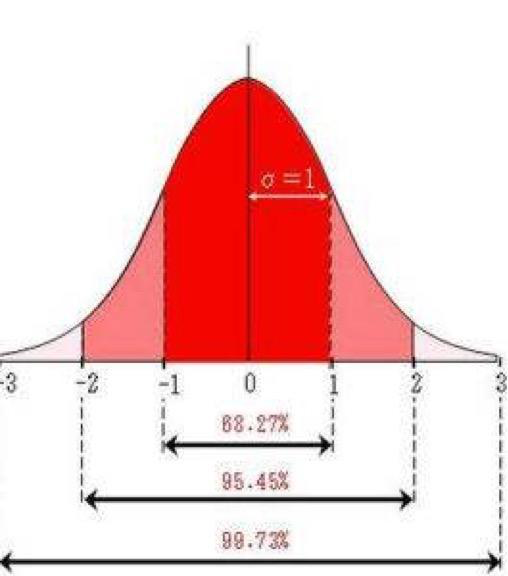一般正态分布 一般正态分布n，假设其均值是 μ，标准差为σ ，即服从 n~N(μ,σ) 经过变换可以转换成标准正态分布：另X = (N - μ)/ σ，则X就是服从标准的正态分布了X~N(0,1)
二、置信区间
上图中的面积就是标准正态分布的概率，而置信区间就是变量的区间估计，例如图中的-1到1就是一个置信区间：标准正态分布的变量X ，有68.27%的概率 X属于[-1,1]这个区间。 最常用的是95%的分布区间，就是[-1.96,1.96]这个区间。方便公式化，我们另区间为[-z,z]，那么 -z<=X<=z。 进而可以推导一般正态分布的置信区间: -z<=X<=z -z<=(N - μ)/ σ<=z μ-zσ<=N<=μ+zσ 因此，一般正态分布n~N(μ,σ)的置信区间是 [μ-zσ, μ+zσ]，其中z根据置信水平而定。置信水平与区间对应关系如下：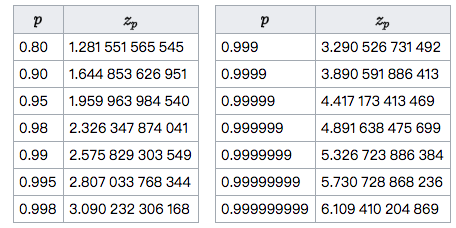性质分析 置信区间与置信水平、样本量等因素均有关系，其中样本量对置信区间的影响为：在置信水平固定的情况下，样本量越多，置信区间越窄。其次，在样本量相同的情况下，置信水平越高，置信区间越宽。 因此：如果样本多，就说明比较可信，不需要很大的修正，所以置信区间会比较窄，下限值会比较大；但是如果样本少，就说明不一定可信，必须进行较大的修正，置信区间会比较宽，下限值会比较小。 由此得出结论：上述正态区间只适用于样本较多的情况，对于小样本，它的准确性很差。
三、威尔逊区间(Wilson score interval)
由于正态区间对于小样本并不可靠，因而，1927年，美国数学家 Edwin Bidwell Wilson提出了一个修正公式，被称为“威尔逊区间”，很好地解决了小样本的准确性问题。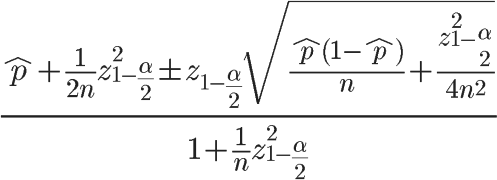在上面的公式中，^p表示样本的”赞成票比例”，n表示样本的大小，z表示对应某个置信水平的z统计量，这是一个常数，可以通过查前文表得到。一般情况下，在95%的置信水平下，z统计量的值为1.96。威尔逊置信区间的均值为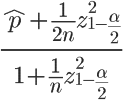下限为：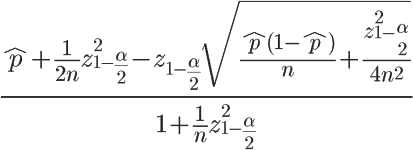可以看到：当n的值足够大时，这个下限值会趋向^p。如果n非常小（投票人很少），这个下限值会大大小于p，实际上，起到了降低”赞成票比例”的作用，使得该项目的得分变小、排名下降。根据离散型随机变量的均值和方差定义： μ=E(X)=0*(1-p)+1*p=p σ=D(X)=(0-E(X))2(1-p)+(1-E(X))2p=p2(1-p)+(1-p)2p=p2-p3+p3-2p2+p=p-p2=p(1-p) 因此上面的威尔逊区间公式可以写成：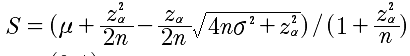就是对正态区间的均值和标准差进行了修正。 但是有个问题：这个修正公式是仅仅适用于伯努利分布（好差评），还是也适用于其他分布（如5星评价）？这个问题本人也没搞清，望高人指点。
计算程序如下：
def wilson_score(pos, total, p_z=2.):
"""
威尔逊得分计算函数
参考：https://en.wikipedia.org/wiki/Binomial_proportion_confidence_interval
:param pos: 正例数
:param total: 总数
:param p_z: 正太分布的分位数
:return: 威尔逊得分
"""
pos_rat = pos * 1. / total * 1.  # 正例比率
score = (pos_rat + (np.square(p_z) / (2. * total))
- ((p_z / (2. * total)) * np.sqrt(4. * total * (1. - pos_rat) * pos_rat + np.square(p_z)))) / \
(1. + np.square(p_z) / total)
return score

tips：对于5星评价问题，可以参考 http://www.evanmiller.org/ranking-items-with-star-ratings.html
展开全文正态分布 伯努利分布
• 根据总体分布（T分布或者Z分布）和规定的置信度计算总体均值在指定置信度下的置信区间，然后将实验值和置信区间比较，若在置信区间之外（小概率事件发生）则表示实验统计量和总体统计量存在显著差异 1.1 总体方差...
目录
1.置信区间的计算
1.1 总体方差已知
1.2 总体方差未知
2.计算 P-Value
2.1 总体方差已知
2.2 总体方差未知

1.置信区间的计算
根据总体分布（T分布或者Z分布）和规定的置信度计算总体均值在指定置信度下的置信区间，然后将实验值和置信区间比较，若在置信区间之外（小概率事件发生）则表示实验统计量和总体统计量存在显著差异
1.1 总体方差已知
总体方差已知时，根据总体均值和方差，使用Z分布计算置信区间，公式如下：

其中：
表示样本均值   表示总体标准差，n表示样本数   表示根据二分之一置信度查表得到的z值， 为显著性水平=1-置信度，若置信度为90%，则  =1-0.9=0.1
1.2 总体方差未知
总体方差未知时，使用样本方差代替总体方差，根据样本方差和总体均值使用T分布计算置信区间，公式如下：

其中：
表示样本均值   表示样本标准差，n表示样本数   表示根据二分之一置信度查表得到的t值， 为显著性水平=1-置信度  n-1是自由度，因为样本均值已知，因此已知n-1个样本，第n个样本就能通过计算得到
注：当样本数量大于30时，T分布和Z分布得到的值十分接近（概率分布图像也十分相似），可以用Z分布代替T分布，换句话说，这时样本方差和总体方差的差距就非常小了
2.计算 P-Value
p值表示当前值或比当前值更极端值出现的概率和，通过和小概率事件（总体统计量分布）的临界值  比较，从而判定样本中的统计量在总体统计量分布中是否属于小概率事件
2.1 总体方差已知
总体方差已知时，根据总体均值和方差，使用Z分布计算P-value，首先要得到z值，z值得计算公式如下：

其中：
为样本均值 为总体均值， 为样本标准差，n为样本数量
得到z值后，查表得到P值，然后设置显著性水平\alpha（\alpha=1-置信度），比如  =0.05，若p值<1- ，则拒绝原假设，样本统计量和总体统计量存在显著性差异，反之则无法拒绝原假设，样本统计量和总体统计量无显著性差异；
2.2 总体方差未知
总体方差未知时，根据总体均值和方差，使用T分布计算P-value，首先要得到t值，t值得计算公式如下：

其中：
为样本均值， 为总体均值，S为样本标准差，n为样本数量
得到t值后，根据自由度（样本数-1）查表得到P值，然后设置显著性水平 （ =1-置信度），比如  =0.05，若p值<1- ，则拒绝原假设，样本统计量和总体统计量存在显著性差异，反之则无法拒绝原假设，样本统计量和总体统计量无显著性差异；
这里显著性水平  本质上就是在总体统计量分布中人为规定一个小概率事件发生的概率；P值就是样本统计量在总体统计量下发生的概率，如果P值< 表明样本统计量在总体统计量的分布中属于小概率事件，因此总体统计量和样本统计量存在显著性差异
参考文章：
假设检验--Z检验、t检验_ws19920726的博客-CSDN博客_z检验公式
置信区间的计算方法_alyssa520的博客-CSDN博客_置信区间计算公式

展开全文假设检验 t检验 z检验
• ## 置信区间的总结

千次阅读 2016-11-06 12:15:04
置信区间的总结@(概率论)置信区间的定义：设θ\theta是总体X的未知参数，X1,X2,...,XnX_1,X_2,...,X_n是来自总体的样本，对于给定的α,(0<α),\alpha,(0 ),如果两个统计量满足：P(θ1<θ<θ2)=1−αP(\theta_1 ) = 1...
置信区间的总结
@(概率论)
置信区间的定义：设

θ
$\theta$是总体X的未知参数，

X1,X2,...,Xn
$X_1,X_2,...,X_n$是来自总体的样本，对于给定的

α,(0<α<1),
$\alpha,(0 < \alpha < 1),$如果两个统计量满足：

P(θ1<θ<θ2)=1−α
$P(\theta_1 < \theta < \theta_2) = 1 - \alpha$, 则称随机区间

(θ1,θ2)
$(\theta_1, \theta_2)$为参数

θ
$\theta$的置信水平为

1−α
$1-\alpha$的置信区间。
注：通常置信度是比较接近于1的数字，因此，

α
$\alpha$是较小的数字。
以一个正态总体分布为例：
设总体

X∼N(μ,σ2),X1,X2,...,Xn
$X\sim N(\mu, \sigma^2), X_1,X_2,...,X_n$是来自总体X的样本，

X⎯⎯⎯
$\overline X$是样本均值，

S2
$S^2$是样本方差。
首先需要明确的是，样本抽出来，则样本均值和样本方差都是已知的了。我们想知道总体的均值和方差是什么，因此，才有了用样本的值去估计总体的选择策略。
也就是说，我们想知道

μ,σ2
$\mu, \sigma^2$是什么，因为是估计，所以得到的是我们有多少把握说这两个值处于一个用样本的观察值表示的区间。
情况可以分为两个大类，
想估计

μ
$\mu$；

σ2
$\sigma^2$已经知道，则这个总体的特征值一定用起来：

X⎯⎯−μσn√∼N(0,1)
$\frac{\overline X - \mu}{\frac{\sigma}{\sqrt n}} \sim N(0,1)$

σ2
$\sigma^2$不知道，那么就需要用样本方差代替：

X⎯⎯−μSn√∼t(n−1)
$\frac{\overline X - \mu}{\frac{S}{\sqrt n}} \sim t(n-1)$估计

σ2
$\sigma^2$：不必管

μ
$\mu$是不是知道，只与样本方差有关系。由浙大版教材的定理二知道：

(n−1)S2σ2∼χ2(n−1)
$\frac{(n-1)S^2}{\sigma^2} \sim \chi^2(n-1)$
待估计的参数有了相应的分布，则代入相应的分布中计算即可。
比如估计

μ
$\mu$，且

σ2
$\sigma^2$已知时：

θ=X⎯⎯⎯−μσn√,θ的置信度是1−αP(θ1<θ<θ2)=1−α;θ1=−U1−α2,θ2=U1−α2;即：P(−U1−α2<θ<U1−α2)=1−α;

\theta = \frac{\overline X - \mu}{\frac{\sigma}{\sqrt n}}, \theta的置信度是1-\alpha \\
P(\theta_1 < \theta < \theta_2) = 1 - \alpha; \\
\theta_1 = -U_{\frac{1-\alpha}{2}},\theta_2 = U_{\frac{1-\alpha}{2}}; \\
即：P(-U_{\frac{1-\alpha}{2}} < \theta < U_{\frac{1-\alpha}{2}}) = 1 - \alpha; \\

由此可得：

μ∈[X⎯⎯⎯−U1−α2⋅σn√,X⎯⎯⎯+U1−α2⋅σn√]
$\mu \in [\overline X-U_{\frac{1-\alpha}{2}}\cdot\frac{\sigma}{\sqrt{n}},\overline X+U_{\frac{1-\alpha}{2}}\cdot\frac{\sigma}{\sqrt{n}}]$
注：

Uα
$U_{\alpha}$指的是

P(X>Uα)=α
$P(X > U_{\alpha}) = \alpha$，也称之为上

α
$\alpha$分位点。
展开全文• 置信区间置信区间Gradient Boosting methods are a very powerful tool for performing accurate predictions quickly, on large datasets, for complex variables that depend non linearly on a lot of features...

置信区间的置信区间

Gradient Boosting methods are a very powerful tool for performing accurate predictions quickly, on large datasets, for complex variables that depend non linearly on a lot of features.
梯度提升方法是一种非常强大的工具，可对大型数据集上的非线性快速依赖许多特征的复杂变量快速执行准确的预测。
Moreover, it has been implemented in various ways: XGBoost, CatBoost, GradientBoostingRegressor, each having its own advantages, discussed here or here. Something these implementations all share is the ability to choose a given objective for training to minimize. And even more interesting is the fact that XGBoost and CatBoost offer easy support for a custom objective function.
而且，它已经以各种方式实现： XGBoost ， CatBoost ， GradientBoostingRegressor ，每种方法都有其各自的优势，在此处或此处进行讨论。 这些实现都具有的共同点是能够选择给定目标进行培训以使其最小化。 更有趣的是，XGBoost和CatBoost为自定义目标函数提供了轻松的支持。
Why do I need a custom objective?
为什么需要自定义目标？
Most implementations provide standard objective functions, like Least Square, Least Deviation, Huber, RMSE, … But sometimes, the problem you’re working on requires a more specific solution to achieve the expected level of precision. Using a custom objective is usually my favourite option for tuning models.
大多数实现都提供标准的目标函数，例如最小二乘，最小偏差，Huber，RMSE等。但是有时，您正在解决的问题需要更具体的解决方案才能达到预期的精度水平。 使用自定义目标通常是我最喜欢的用于调整模型的选项。
Can you provide us with an example?
您能提供一个例子吗？
Sure! Recently, I’ve been looking for a way to associate the prediction of one of our models with confidence intervals. As a short reminder, confidence intervals are characterised by two elements:
当然！ 最近，我一直在寻找一种将我们模型之一的预测与置信区间相关联的方法。 简要提醒一下，置信区间的特征包括两个要素：
An interval [x_l, x_u] 间隔[x_l，x_u] The confidence level i.e. the probability that the predicted values lie in this interval. 置信度， 即预测值在此间隔内的概率。
For instance, we can say that the 99% confidence interval of average temperature on earth is [-80, 60].
例如，我们可以说地球上平均温度的99％置信区间为[-80，60]。
Associating confidence intervals with predictions allows us to quantify the level of trust in a prediction.
将置信区间与预测相关联可以使我们量化预测中的信任级别。
How do you compute confidence intervals?
您如何计算置信区间？
You’ll need to train two models :
您需要训练两个模型：
One for the upper bound of your interval 一个为间隔的上限 One for the lower bound of your interval 一个用于间隔的下限
And guess what? You need specific metrics to achieve that: Quantile Regression objectives. Both the scikit-learn GradientBoostingRegressor and CatBoost implementations provide a way to compute these, using Quantile Regression objective functions, but both use the non-smooth standard definition of this regression :
Where t_i is the ith true value and a_i is the ith predicted value. w_i are optional weights used to ponderate the error. And alpha defines the quantile.
其中t_i是第i个真实值，而a_i是第i个预测值。 w_i是用于考虑错误的可选权重。 alpha定义了分位数。
For instance, using this objective function, if you set alpha to 0.95, 95% of the obervations are below the predicted value. Conversely, if you set alpha to 0.05, only 5% of the observations are below the prediction. And 90% of real values lie between these two predictions.
例如，使用此目标函数，如果将alpha设置为0.95，则95％的观测值低于预测值。 相反，如果将alpha设置为0.05，则只有5％的观测值低于预测值。 90％的实际价值介于这两个预测之间。
Let’s plot it using the following code, for the range [-10, 10] and various alphas:
让我们使用以下代码为[-10，10]范围和各种alpha进行绘制：

As you can see in the resulting plot below, this objective function is continuous but its derivative is not. There is a singularity in (0, 0), i.e. it’s a C_0 function, with respect to the error, but not a C_1 function. This is an issue, as gradient boosting methods require an objective function of class C_2, i.e. that can be differentiated twice to compute the gradient and hessian matrices.
正如您在下面的结果图中看到的那样，该目标函数是连续的，但其导数不是。 (0，0)中有一个奇点， 即 。 关于错误，它是一个C_0函数，但不是C_1函数。 这是一个问题，因为梯度增强方法需要C_2类的目标函数，即可以将其微分两次以计算梯度和粗麻布矩阵。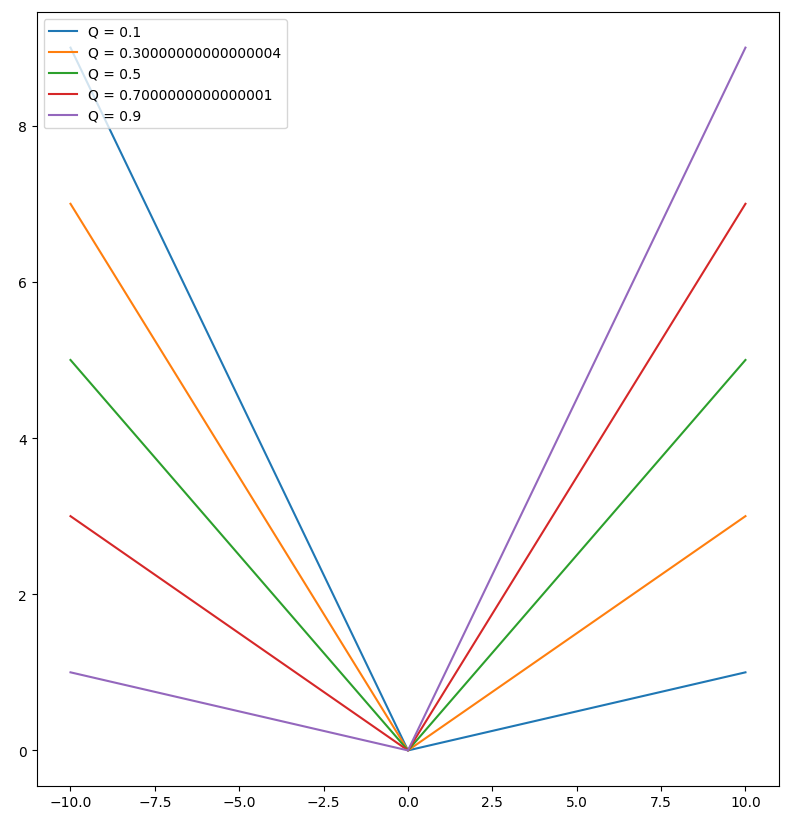If you are familiar with the MAE objective, you should have recognized that these quantile regression functions are simply the MAE, scaled and rotated. If you’re not, the screenshot below should convince you :
如果您熟悉MAE目标，那么您应该已经认识到这些分位数回归函数就是简单的MAE，可以对其进行缩放和旋转。 如果不是这样，下面的屏幕截图应该可以说服您：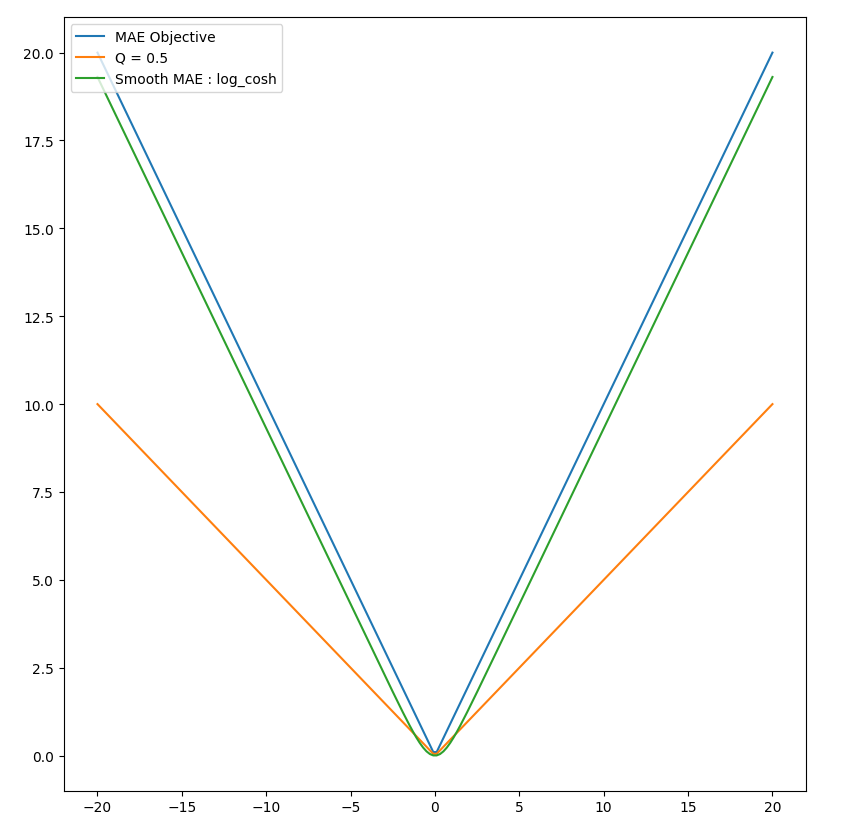The logcosh objective
逻辑目标
As a reminder, the formula for the MAE objective is simply
提醒一下，MAE目标的公式很简单
MAE objective formula

The figure above also shows a regularized version of the MAE, the logcosh objective. As you can see, this objective is very close to the MAE, but is smooth, i.e. its derivative is continuous and differentiable. Hence, it can be used as an objective in any gradient boosting method, and provides a reasonable rate of convergence compared to default, non-differentiable ones.
上图还显示了MAE(logcosh目标)的正规化版本。 如您所见，该目标非常接近MAE，但很平滑，即其导数是连续且可微的。 因此，它可以用作任何梯度增强方法的目标，并且与默认的不可微方法相比，可以提供合理的收敛速度。
And as it is a very close approximation of the MAE, if we manage to scale and rotate it, we’ll get a twice differentiable approximation of the quantile regression objective function.
而且由于它是MAE的非常接近的近似值，因此，如果我们能够缩放和旋转它，我们将得到分位数回归目标函数的二次微分近似值。
You might have noticed that there is a slight offset between the curve of the MAE and the log cosh. We will explain that in detail a little further below.
您可能已经注意到，MAE的曲线和对数曲线之间存在一些偏移。 我们将在下面进一步详细解释。
The formula for the logcosh is straightforward :
Logcosh的公式很简单：
Formula for the logcosh objective

Rotation and scaling of the logcosh
Logcosh的旋转和缩放
All we need to do now is to find a way to rotate and scale this objective so that it becomes a good approximation of the quantile regression objective. Nothing complex here. As logcosh is similar to the MAE, we apply the same kind of change as for the Quantile Regression, i.e. we scale it using alpha :
我们现在要做的就是找到一种旋转和缩放该目标的方法，以使其成为分位数回归目标的良好近似。 这里没什么复杂的。 由于logcosh与MAE相似，因此我们采用与分位数回归相同的更改，即我们使用alpha对其进行缩放：
Smooth Quantile regression using log cosh

That can be done with these twelve lines of code:
这可以通过以下十二行代码来完成：

And this works, as shown below :
这可以正常工作，如下所示：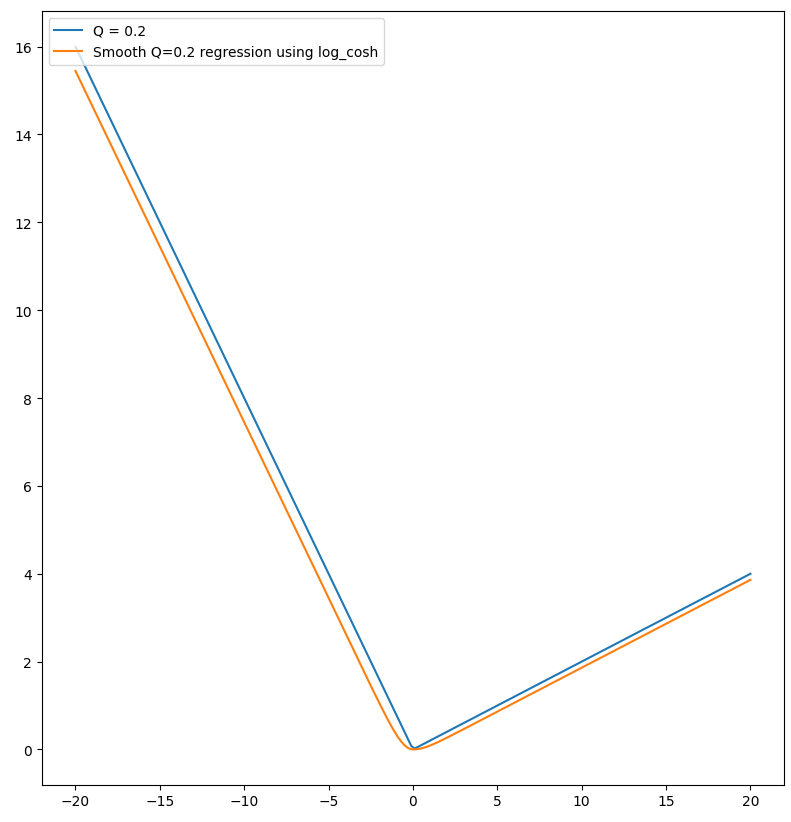But wait a minute!
但是请稍等！
You might be curious as to why combining two non-linear functions like log and cosh results in such a simple, near linear curve.
您可能对为什么将两个非线性函数(例如log和cosh)组合在一起产生如此简单，接近线性的曲线感到好奇。
The answer lies in the formula of cosh :
答案在于cosh的公式：
cosh formula

When x is positive and large enough, cosh can be approximated by
当x为正且足够大时， cosh可以近似为
Approximation of cosh when x >> 0

Conversely, when x is negative enough, cosh can be approximated by
相反，当x足够负时， cosh可以近似为
Approximation of cosh when x << 0

We begin to understand how combining these two formulae leads to such linear results. Indeed, as we apply the log to these approximations of cosh, we get :
我们开始理解将这两个公式结合起来如何得出这样的线性结果。 实际上，将对数应用于这些近似的cosh时，我们得到：
logcosh simplification for x >> 0

for x >>0. The same stands for x << 0 :
对于x >> 0。 x << 0的相同含义：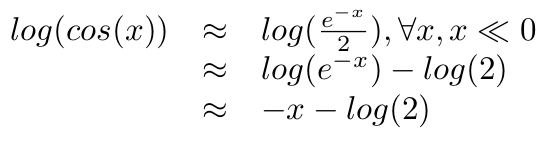It is now clear why these two functions closely approximate the MAE. We also get as a side benefit the explanation for the slight gap between the MAE and the logcosh. It’s log(2)!
现在很清楚，为什么这两个函数非常接近MAE。 作为附带的好处，我们还可以解释MAE和Logcosh之间的微小差距。 是log(2)！

Let’s try it on a real example
让我们尝试一个真实的例子
It is now time to ensure that all the theoretical maths we perform above works in real life. We won’t evaluate our method on a simple sinus, as proposed in scikit here ;) Instead, we are going to use real-world data, extracted from the TLC trip record dataset, that contains more than 1 billion taxi trips.
现在是时候确保我们在现实生活中完成以上工作的所有理论数学。 我们不会在这里的 scikit中提出的那样对基于简单窦的方法进行评估；)相反，我们将使用从TLC行程记录数据集中提取的包含10亿次出租车行程的真实数据。
The code snippet below implements the idea presented above. It defines the logcosh quantile regression objective log_cosh_quantile, that computes its gradient and the hessian. Those are required to minimize the objective.
下面的代码片段实现了上面介绍的想法。 它定义了logcosh分位数回归目标log_cosh_quantile ，该目标计算其梯度和粗麻布。 需要这些以最小化目标。
As stated at the beginning of this article, we need to train two models, one for the upper bound, and another one for the lower bound.
如本文开头所述，我们需要训练两个模型，一个模型用于上限，另一个模型用于下限。
The remaining part of the code simply loads data and performs minimal data cleaning, mainly removing outliers.
该代码的其余部分仅加载数据并执行最少的数据清理，主要是消除异常值。

In this code, we have chosen to compute the 90% confidence interval. Hence we use alpha=0.95 for the upper bound, and alpha=0.05 for the lower bound.
在此代码中，我们选择了计算90％置信区间。 因此，我们将alpha = 0.95用作上限，将alpha = 0.05用作下限。
Hyperparameter tuning has been done manually, using fairly standard values. It could certainly be improved, but the results are good enough to illustrate this paper.
超参数调整已使用相当标准的值手动完成。 当然可以改进它，但是结果足以说明本文。
The last lines of the script are dedicated to the plotting of the first 150 predictions of the randomly build test set with their confidence interval:
脚本的最后几行专门用于绘制随机构建测试集的前150个预测及其置信区间：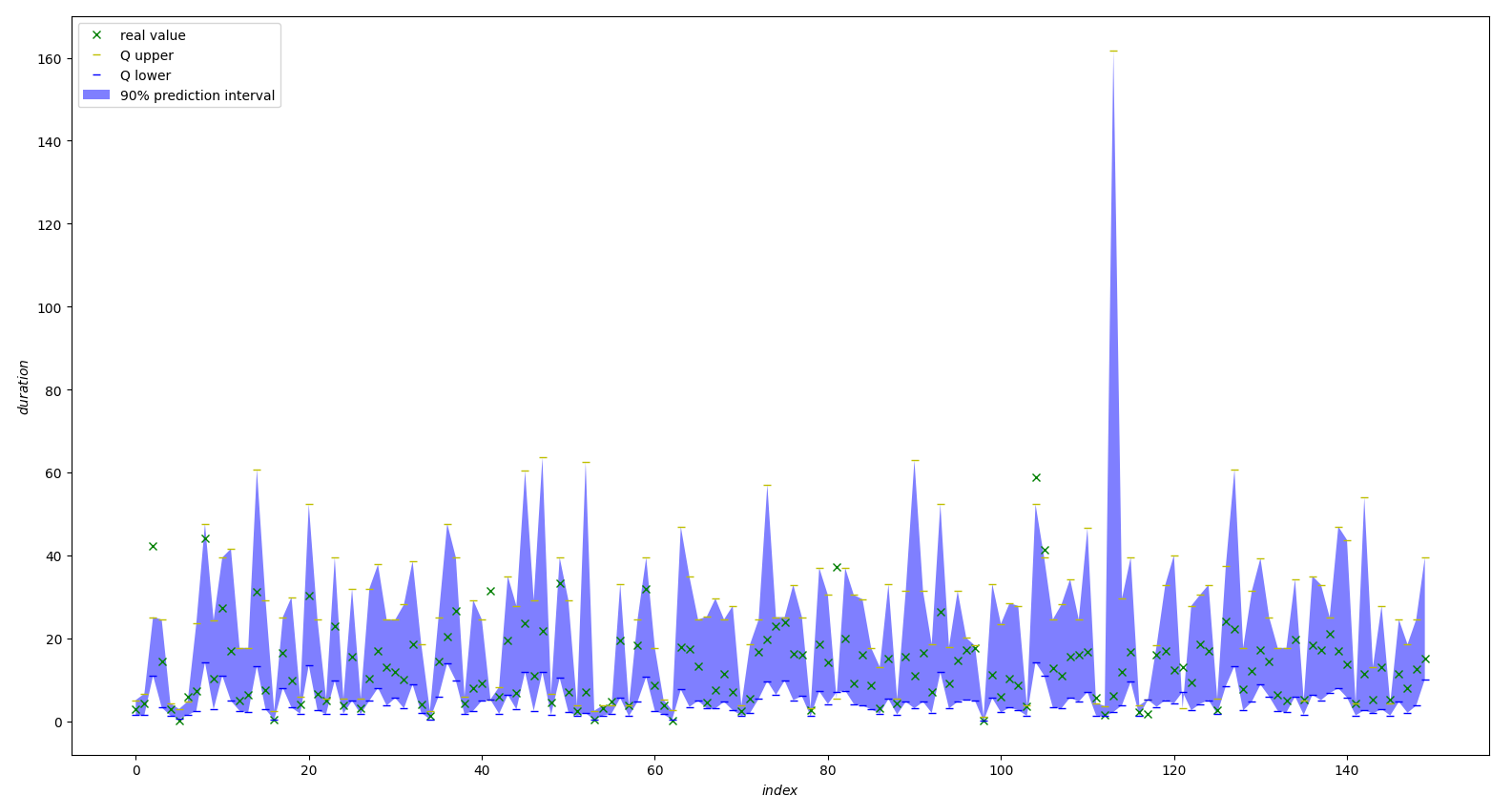Note that we have also included at the end of the script a counter to evaluate the number of real values whose confidence interval is correct. On our test set, 22 238 over 24 889 (89.3%) of the real values were within the calculated confidence interval.
注意，在脚本的末尾还包含一个计数器，用于评估置信区间正确的实数值的数量。 在我们的测试集中，超过24 889的实际值中的22 238(89.3％)在计算的置信区间内。
The model has been trained on the first 100 000 lines of the January 2020 dataset of the TLC trip record dataset.
该模型已在TLC旅行记录数据集的2020年1月数据集的前100000行上进行了训练。
Conclusion
结论
With simple maths, we have been able to define a smooth quantile regression objective function, that can be plugged into any machine learning algorithm based on objective optimisation.
通过简单的数学运算，我们已经能够定义一个平滑的分位数回归目标函数，该函数可以插入基于目标优化的任何机器学习算法中。
Using these regularized functions, we have been able to predict reliable confidence intervals for our prediction.
使用这些正则化函数，我们已经能够为我们的预测预测可靠的置信区间。
This method has the advantage over the one presented here of being parameters-less. Hyperparameter tuning is already a demanding step in optimizing ML models, we don’t need to increase the size of the configuration space with another parameter ;)
与此处介绍的方法相比，此方法的优点在于无需参数。 在优化ML模型中，超参数调整已经是一个艰巨的步骤，我们不需要使用其他参数来增加配置空间的大小；)

翻译自: https://towardsdatascience.com/confidence-intervals-for-xgboost-cac2955a8fde

置信区间的置信区间

展开全文python java
• μ=5,θ=4,n=10,K=100,α=0.1\mu = 5,\theta = 4,n = 10, K = 100...CI_U<-NULL for (k in 1:100){ samps<-rnorm(10,mean=5,sd=2) mu<-mean(samps) sd<-sd(samps) critv<-qt(1-0.1/2,9) CI_L<机器学习 算法 聚类算法
• 1.点估计与区间估计 首先我们看看点估计的含义： 是用样本统计量来估计总体参数，因为样本...给定置信水平，根据估计值确定真实值可能出现的区间范围，该区间通常以估计值为中心，该区间则为置信区间。 2.中心极限...
• 目录 1： 枢轴量 ... 求出置信水平为的置信区间 1.2 方法： 1： 随机变量G,G的分布已知 2： 找到a<b,使得 G是关于和样本的函数 3： 从a<G<b ,解出 就是参数的双侧置信区...
• ## 95% 置信区间

千次阅读 2020-08-31 10:19:33
95%置信区间是一个随即的区间，就是指端点为随机变量，这个随即变量通常是一个统计量，当抽取不同的样本时就对应不同的值，从而对应不同的区间。对于某些样本来说，对应的区间包含参数的真值，另一些不包含。若早100...
• 假设有一个总体很大，我们共调查了nnn个人，其中持有某种观点的为xxx人，则样本比例为p^=x/n\hat {p}=x/np^​=x/n,那么比例ppp的100(1−α)%100(1-\alpha) \%100(1−α)%近似置信区间为： p^±zα/2p^(1−p^)n \hat{...R语言
• 二项分布计算置信区间有多种计算公式，最常见的是“正太区间”（Normal approximation interval），但它只适用于样本较多的情况（np > 5 且 n(1 − p) > 5），对于小样本，它的准确性很差。Wilson算法正是解决...
• ## 单侧置信区间

千次阅读 2019-05-26 09:49:29
• 我们知道参数的置信区间的计算，这些都服从一定的分布(t分布、正态分布），因此在标准误前乘以相应的t分值或Z分值。但如果我们找不到合适的分布时，就无法计算置信区间了吗？幸运的是，有一种方法几乎可以用于计算...R语言 Bootstrap 线性回归
• 在点估计的基础上，从抽样数据的统计计算中可以对其与总体样本的真实参数的接近程度求出一个概率度量，在此概率下给出总体参数估计的一个可信区间范围。（区间估计） 这类问题就被统称为参数估计问题。 一、...参数估计 大数定律
• 尽力搞懂统计学概念——点估计、区间估计、 置信区间 在统计学的应用过程中，总有那么几个重要的基础概念似懂非懂，就像雾里看花，你对它有一个总体的印象，但说道具体细节又似是而非。我也深受其扰，现在就结合...
•   在假设检验中，我们常常看到跟P值形影不离的一对区间值， 就是大名鼎鼎的置信区间了。 这置信区间和P值是怎么得来的，我想大多数盆友都不会有什么直观的概念，只会注意P值是否小于0.05或者0.01(根据显著性水平...p值
• R代码，关于伽马分布中参数的广义置信区间及其覆盖率，论文不好复制这里，广义置信区间的代码都可以参考一下。 GCIab<- function(a,b,n,nr,alpha){ y <- rgamma(n,shape = a,scale = b) x1<- y^(1/3) x1...r语言
• 指定置信区间的随机数生成器请注意，它使用高斯Z作为种子，而不使用通常的统一U来促进相关变量的生成 •• • 例子 import { norm , logn } from 'grosso-modo' const n = norm ( 2 , 4 ) ( ) , // normal ...JavaScript
• confidence intervals1.基本概念1.1 统计推断 statistical inferences1.2 估计量和估计1.3进行...置信区间3.1 z-interval3.2 t interval3.3 p的的z区间:4.Determining Sample Size4.1determining sample size - μ4.统计学
• （1）计算认为有必要的概率是99%的置信区间。 （2）在保证概率是99%的前提下该如何缩小置信区间 （1）分析：只有两种情况，一种是有必要，另一种是没必要。是伯努利分布。假设不必要是0，发生概率是1-p。必要是1，...
• 在上一课中，我们讨论了使用引导程序来获得预测的置信区间的方法。我把正在进行的在线输入代码（很简单的评论，我可以回到旧票ACT6420课程补充剂）。我们将在我最喜欢的基础上讨论线性回归（在讨论配置三角形之前，...R语言 回归模型
• 对于置信区间一直不了解，很难懂，哪位大神可以通俗易懂地解释一下，最好举个例子，谢谢！ 关注者 3,198 被浏览 456,107 关注问题写回答 ​3 条评论 ​分享 ​邀请回答 ​ 85 个回答 默认排序​ 马...
• 这里就涉及到统计学里面的置信区间置信度，本文简要介绍了置信区间这一核心概念，它有助于我们从直观上理解评价估计优劣的度量方法。 本文讨论了统计学中的一个基本术语 ：置信区间。我们仅以一种非常友好的方式...统计学
• 转自：机器之心Pro，《入门 | 我们常听说的置信区间置信度到底是什么？》作者：Dima Shulga 参与：程耀彤、思源 原文地址：https://www.sohu.com/a/226540397_129720 一、引用正文： 机器学习本质上是对条件概率...机器学习 统计学
• ## Python 计算置信区间

万次阅读 2015-01-16 16:17:02
Python 计算置信区间 用Python实现http://vassarstats.net/prop1.html 所计算的置信区间 import math #计算置信区间的函数 def calc(r,n): if n print ('r cannot be greater than n.') return if ...Python
• 区间估计简介Python求解单个正态总体参数的置信区间参考区间估计简介假定参数是射击靶上 10 环的位置，作一次射击，打在靶心 10 环的位置上的可能性很小，但打在靶子上的可能性就很大，...python 深度学习 统计学
• 名称或 L95 U95， 生成可用于发布的森林图，并将结果保存为 .fig 以供手动调整和高分辨率 .png .. 此功能还可以处理使用一行 NA 拆分子标题下的森林图。 有关详细信息，请参阅示例。 包含使用线性或对数标度绘图的...matlab
• 四、置信区间上界算法 In : import math N = 10000 # 1000个用户 d = 10 # 10个广告 ads_selected = [] # 广告选择 numbers_of_selections = [ 0 ] * d # 多项选择 ...算法 机器学习
• #sigma已知u的1-α置信区间 duishuci1 <-function(n,mu,sigma,alpha) { x=rlnorm(n,mu,sigma) shuzhou <-sum(log(x))/n mucimin <-shuzhou-sigma*(n**(-1/2))*qnorm(alpha/2,0,1,lr语言
• 带有置信区间的条形图 library(gplots) attach(airquality) heights <- tapply(Temp,Month,mean) lower <- tapply(Temp,Month,function(v) t.test(v)\$conf.int) upper <- tapply(Temp,Month,function(v)...可视化 R
• ## 置信区间，统计量

千次阅读 2018-06-10 11:57:11
关键字：置信区间1.作用：描述一个区间有多大的概率包含未知参数2.定义 设母体的概率函数为f(x;seta)，seta为未知参数，有一个取自母体的子样，字样有n个数据。如果对事先给定的a，0&lt;a&lt;1，存在两个......